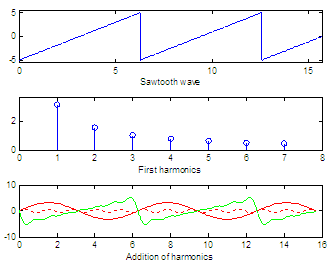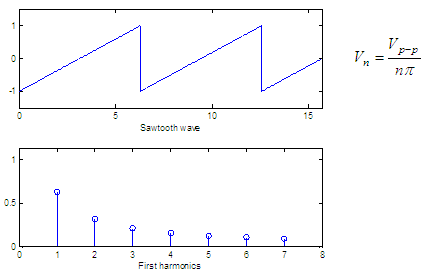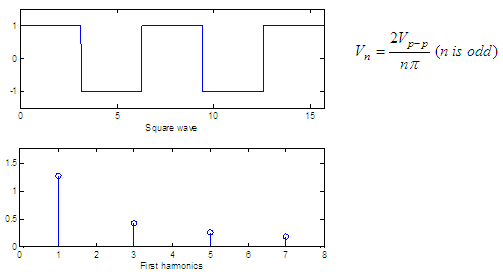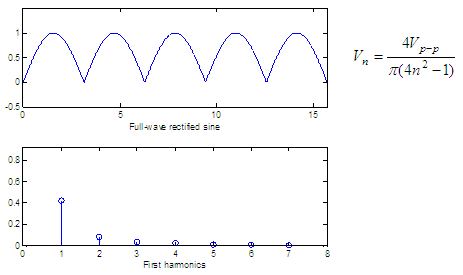Fourier Series - an understandable introduction...

In mathematics, the Fourier series is an infinite sequence of terms used to solve special types of problems.

The series consists of an infinite sum of sines and cosines that repeats over fixed intervals, and so is very useful for analyzing periodic functions.

Fourier theorem is the key to the analysis in the frequency domain when talking about electronic applications.

According to the Fourier theorem, a wave or signal is composed of a series of sinusoidal components whose frequencies are those of the fundamental freq. and its harmonics, each component having the proper amplitude and phase. The sequence of components that form this wave is called its spectrum.

There are, at least, two ways to perform a circuit analysis. First, you can find what happens with the periodic waveform at every instant of time; second, you can determine what happens to each of its harmonics. Sometimes, the first analysis (time domain) is fast, but often the second analysis (frequency domain) is better.

Spectral components

Suppose Vp-p represents the peak-to-peak value of a sawtooth wave. It can be demonstrated that the amplitude of each harmonic is

Vn = Vp-p /(nπ)

This means that the peak value of the nth harmonic is equal to Vp-p divided by nπ.

For example, the top subplot in this figure shows a sawtooth wave with a peak-to-peak value of 10,the harmonics have then the following peak values:

V1 = 10/(1π) = 3.18
V2 = 10/(2π) = 1.59
V3 = 10/(3π) = 1.06

The second subplot in the figure above shows the first seven magnitudes of the respective harmonics. The bottom subplot shows how the addition of different harmonics (red lines) can produce something close to the original sawtooth (green line): the more considered terms the more accuracy.

You can learn some ideas on how to produce periodic square or sawtooth waves, here.

The Matlab code to produce the first two subplots in the figure above, is:

x = 0 : .01 : 5*pi;
y = 5 * sawtooth(x);
subplot(311), plot(x, y)
axis([min(x) max(x) min(y)-.5 max(y)+.5])
xlabel(
'Sawtooth wave')

n = 1 : 7;
v = 10 ./(n*pi);
subplot(312), stem(n, v)
axis([min(n)-1 max(n)+1 0 max(v)+.5])
xlabel(
'First harmonics')

With an oscilloscope (time domain tool) you can see the periodic signal as a function of time. The vertical axis represents voltage and the horizontal axis represents time, in fact, the oscilloscope represents the instantaneous value V of the periodic wave.

The spectrum analyzer is different because it’s an instrument of the frequency domain. The horizontal axis represents the frequency, and the vertical axis represents the amplitude of the harmonics. For example, you would use an oscilloscope to observe the top subplot above. You’d use a spectrum analyzer to observe the middle subplot above (that's what the Fourier series are for). This kind of information is called the spectrum, where each level of the vertical lines represents the peak value of the harmonics, and the horizontal axis shows the corresponding frequency.

Any periodic wave has a spectrum or a set of vertical lines representing the harmonics. The spectrum naturally differs from one periodic signal to another.

Three basic spectra

The figures below show three periodic waves and their spectra. In each case, Vp-p is the peak-to-peak value of the periodic wave and for convenience we show only the harmonics up to n = 7. The formula of the harmonic peak appears for each case.You can learn some ideas on how to produce square and sawtooth functions, here.

The code to produce the Fourier series of harmonics above is:

clear, clc, close all

x = 0 : .01 : 5*pi;
y = sawtooth(x);
subplot(211), plot(x, y)
axis([min(x) max(x) min(y)-.5 max(y)+.5])
xlabel(
'Sawtooth wave')

n = 1 : 7;
v = 2 ./(n*pi);
subplot(212), stem(n, v)
axis([min(n)-1 max(n)+1 0 max(v)+.5])
xlabel(
'First harmonics')

figure
y = square(x);
subplot(211), plot(x, y)
axis([min(x) max(x) min(y)-.5 max(y)+.5])
xlabel(
'Square wave')

n = 1 : 2 : 7;
v = 4 ./(n*pi);
subplot(212), stem(n, v)
axis([min(n)-1 max(n)+1 0 max(v)+.5])
xlabel(
'First harmonics')

figure
y = abs(sin(x));
subplot(211), plot(x, y)
axis([min(x) max(x) min(y)-.5 max(y)+.5])
xlabel(
'Full-wave rectified sine')

n = 1 : 7;
v = 4 ./(pi*(4*n.^2 - 1));
subplot(212), stem(n, v)
axis([min(n)-1 max(n)+1 0 max(v)+.5])
xlabel(
'First harmonics')

Reference:
Malvino, A. P.; Electronic Principles; 2nd. edition; McGraw-Hill Co., 1979.

From ' Fourier Series ' to home

From ' Fourier Series ' to Calculus Problems

 Top Fourier intro Periodic Functions Harmonic Series